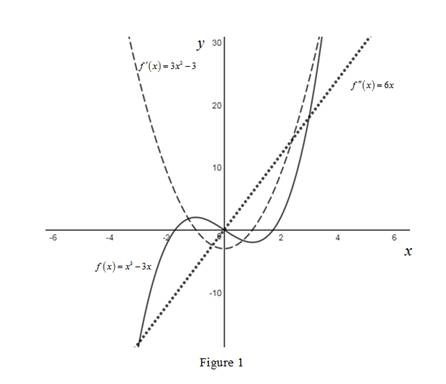# The first and second derivative of the function f ( x ) = x 3 − 3 x and sketch its graphs.### Single Variable Calculus: Concepts...

4th Edition
James Stewart
Publisher: Cengage Learning
ISBN: 9781337687805### Single Variable Calculus: Concepts...

4th Edition
James Stewart
Publisher: Cengage Learning
ISBN: 9781337687805

#### Solutions

Chapter 2.7, Problem 46E
To determine

## To find: The first and second derivative of the function f(x)=x3−3x and sketch its graphs.

Expert Solution

The first and second derivatives are, f(x)=3x23 and f(x)=6x.

### Explanation of Solution

Given:

The function is, f(x)=x33x.

Formula used:

The derivative of a function f , denoted by f(x), is

f(x)=limh0f(x+h)f(x)h (1)

Calculation:

Obtain the first derivative of the function f(x).

Compute f(x) by using the equation (1),

f(x)=limh0f(x+h)f(x)h=limh0(x+h)33(x+h){x33x}h=limh0x3+h3+3x2h+3xh23x3hx3+3xh

Simplify the numerator and obtain the derivative of the function,

f(x)=limh0h3+3x2h+3xh23hh=limh0h(h2+3x2+3xh3)h

Since the limit h approaches zero but is not equal to zero, cancel the common term h from both the numerator and the denominator,

f(x)=limh0(h2+3x2+3xh3)=02+3x2+3x(0)3=3x23

Thus, the first derivative of the function is, f(x)=3x23.

Obtain the second derivative of the function f(x).

Compute f(x) by using the equation (1),

f(x)=(f(x))=limh0f(x+h)f(x)h=limh03(x+h)23{3x23}h=limh03x2+3h2+6xh33x2+3h

Simplify the numerator,

f(x)=limh03h2+6xhh=limh0h(3h+6x)h

Since the limit h approaches zero but is not equal to zero, cancel the common term h from both the numerator and the denominator,

f(x)=limh0(3h+6x)=3(0)+6x=6x

Thus, the second derivative of the function is, f(x)=6x.

Therefore, the first and second derivatives of the functions are, f(x)=3x23 and f(x)=6x.

Use the online graphing calculator to draw the graph of the functions f(x),f(x) and f(x) as shown below in Figure 1.From Figure 1, it is observed that the graph of f(x) is a curve and the graph of f(x) is a straight line.

Take several points on the domain and estimate the slope of the function f(x) which is equal to the value of f(x) at that point.

For example, take the point 1 and the value of the slope of the tangent to the function f(x) at 1 is 0 (horizontal tangent) which is same as the value f(1)=0.

Thus, the derivative f(x) is reasonable.

Take several points on the domain and estimate the slope of tangent to the function f(x) which is equal to the value of f(x) at that point.

For example take the point 2 and the value of the slope of the tangent to the function f(x) at 2 is 12 which is same as the value f(2)=12.

Thus, the derivative f(x) is reasonable.

### Have a homework question?

Subscribe to bartleby learn! Ask subject matter experts 30 homework questions each month. Plus, you’ll have access to millions of step-by-step textbook answers!